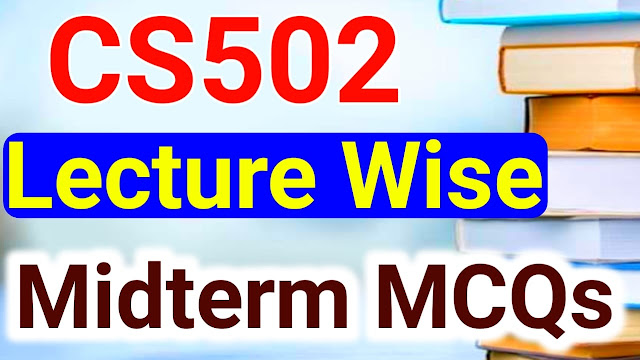# CS502 Lecture Wise MCQs Midterm

### CS502 Lecture Wise Solved MCQs

 Post Title CS502 Lecture Wise MCQs Midterm Book Code CS502 Degree BSIT/BSCS/Other University Virtual Univerity of Pakistan CS502 Quiz 1/ Quiz 2 / Quiz 3 CS502 Quiz 1 Mid/Final CS502 Lecture Wise MCQs Midterm Also, Read CS401 Solved MCQs Midterm Also, Read CS601 Solved MCQs Midterm

### CS502 Lecture Wise MCQs Midterm

In ram, each instruction involves performing some basic operation on how many values are in the machine's memory?  (CS502)

One

Three

Four

Two

The ………..is the weighted sum of running times with weights.  (CS502)

Average case time

Best case time

Both best case and average case

Worse case time

Merge sort is based on ………..(CS502)

Axis sweep

Divide and conquer

Brute force

Pian sweep

In the following code the statement ‘cout << i,’ executes …………. Times for [int i = 1, i < = n; i++] cout<<i;  (CS502)

Infinite times

N+5 times

N times

Zero times

The main purpose of mathematical analysis is to measure the ………required by the algorithm.  (CS502)

Space

Execution time and memory

Inputs & outputs

Execution time

The important factors to measure the running time of the brute force 2-d maxima algorithm is.  (CS502)

Count the number of times an element of P is accessed

Count the number of steps of the pseudo-code that is executed

All of above

The number of comparisons that are performed

Pseudocode of algorithms are to be read by…………(CS502)

Computer

Compiler

People

RAM

If input ‘n’ is odd, then the median will be …………..(CS502)

(n-1)/2

(n+1)/2

N+2

n/2

Merge sort is based on ………..(CS502)

Axis sweep

Divide and conquer

Pian sweep

Brute force

The cubic function will………..a quadratic function.  (CS502)

Find

Prove

Be equal to

Overtake

Merge sort algorithm discussed in handouts contains………(CS502)

One loop only

4 loops

2 loops

3 loops

Using ……………..algorithm, efficiency is not given much importance.  (CS502)

Processing

Merge sort

Greedy

Brute force

In the statement if (p[i].x & (p[i].y < p[j].y): the number of times elements of p are accessed is ………..(CS502)

4

1

2

3

For small values of n ………… the algorithm is fast enough.   (CS502)

Quicksort

Heapsort

Merge sort

Any

For solving the selection problem, we introduced the sieving technique due to …………  (CS502)

Eliminating rank of an element

Avoiding to sort all input data

Using brute force approach

Using decrease and conquer strategyCS502 Lecture Wise MCQs Midterm